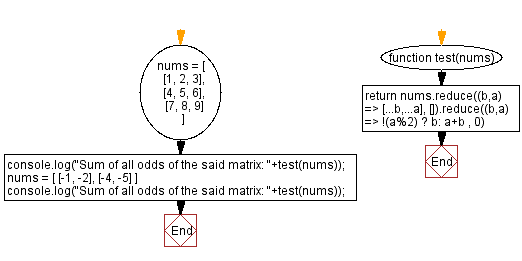# JavaScript: Sum of all odds in a matrix

## JavaScript Math: Exercise-91 with Solution

Write a JavaScript program to calculate the sum of all odd elements in a square matrix.

Test Data:
([ [1, 2, 3], [4, 5, 6], [7, 8, 9] ]) -> 25
( [ [-1, -2], [-4, -5] ]) -> -6

Sample Solution:

HTML Code:

``````<!DOCTYPE html>
<html>
<meta charset="utf-8">
<title>JavaScript program to Sum of all odds in a matrix</title>
<body>

</body>
</html>
```
```

JavaScript Code:

``````function test(nums) {
return nums.reduce((b,a) => [...b,...a], []).reduce((b,a) => !(a%2) ? b: a+b , 0)
}
nums = [
[1, 2, 3],
[4, 5, 6],
[7, 8, 9]
]
console.log("Sum of all odds of the said matrix: "+test(nums));
nums = [
[-1, -2],
[-4, -5]
]
console.log("Sum of all odds of the said matrix: "+test(nums));

```
```

Sample Output:

```Sum of all odds of the said matrix: 25
Sum of all odds of the said matrix: -6
```

Flowchart:Live Demo:

See the Pen javascript-math-exercise-91 by w3resource (@w3resource) on CodePen.

Improve this sample solution and post your code through Disqus

What is the difficulty level of this exercise?

Test your Programming skills with w3resource's quiz.

﻿